• ## 文法

2020-04-30 15:36:25
文法 α->ϐ;α必须含有大写 非终结符：大写，可以拆 终结符：小写，不可以拆 文法类型 0型文法 VN ：非终结符集 VT ：终结符集 P ：产生式集合（规则集合） S ：开始符号（识别符号） α∈(VN∪VT)*：VN和VT 的...
文法
α->ϐ;α必须含有大写 非终结符：大写，可以拆 终结符：小写，不可以拆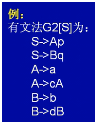文法类型
0型文法
VN ：非终结符集 VT ：终结符集 P ：产生式集合（规则集合） S ：开始符号（识别符号） α∈(VN∪VT)*：VN和VT 的所有元素的随机组合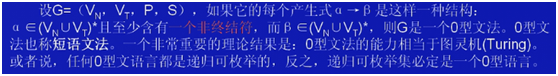结论：1.α∈(VN∪VT)* ，且至少含一个VN（大写），β∈(VN∪VT)* 2.0型文法的能力相当于图灵机（Turing）。或者说，任何0型语言都是递归可枚举的；反之，递归可枚举集必定是一个0型语言。
1型文法（上下文有关文法）
|β|：β的字符串长度 |α|：α的字符串长度 |β|>=|α| 例如：B->Aa(符合)Aa->b(不符合)
2型文法（上下文无关文法）
1型文法基础上，α必须是非终结符 例如 Ab=abc（不符合，属于一型文法但是α= Ab时终结符）
3型文法（正规文法）
第一点：左边必须只有一个字符，且必须是非终结符； 第二点：其右边最多只能有两个字符，且当有两个字符时必须有一个为终结符而另一个为非终结符。当右边只有一个字符时，此字符必须为终结符。 第三点：对于3型文法中的所有产生式，其右边有两个字符的产生式，这些产生式右边两个字符中终结符和非终结符的相对位置一定要固定，也就是说如果一个产生式右边的两个字符的排列是：终结符＋非终结符，那么所有产生式右边只要有两个字符的，都必须前面是终结符而后面是非终结符。反之亦然，要么，就全是：非终结符＋终结符。 每个产生式均为 “A→aB”或“A→a” —— 右线性 　　 “A→Ba”或“A→a” —— 左线性
展开全文• 0型文法、1型文法、2型文法、3型文法
引用：  ＝＝＝＝＝＝＝＝＝＝＝＝＝＝＝＝＝＝＝＝  S-> aaS|a是什么型的，为什么  S-> aSb|ab是什么型的，为什么  S-> SaS|b是什么型的，为什么  ＝＝＝＝＝＝＝＝＝＝＝＝＝＝＝＝＝＝＝＝＝   答：三种文法都属于上下文无关文法。   四种文法的判断非常简单，说到到，四种文法就是规定产生式的左和右边的字符的组成规则不同而已，其它的不能理解就不要去想了，你只要知道判断的时候就是以产生式的左边和右边符合的规则进行判断。下面解释一下如何根据产生式左边和右边的特征来进行判断。   首先，应该明确，四种文法，从0型到3型，其规则和约定越来越多，限制条件也越来越多，所以，我们判断时可以从最复杂的3型进行判断，依次向下判断，如果不符合3型的，那再看是不是2型的，不是2型的，再看是不是1型的，当然，对于作题作的熟的朋友，不用这么复杂，可以一眼直接看出来。   3型文法遵循什么规范呢？  第一点：左边必须只有一个字符，且必须是非终结符；  第二点：其右边最多只能有两个字符，且当有两个字符时必须有一个为终结符而另一个为非终结符。当右边只有一个字符时，此字符必须为终结符。  第三点：对于3型文法中的所有产生式，其右边有两个字符的产生式，这些产生式右边两个字符中终结符和非终结符的相对位置一定要固定，也就是说如果一个产生式右边的两个字符的排列是：终结符＋非终结符，那么所有产生式右边只要有两个字符的，都必须前面是终结符而后面是非终结符。反之亦然，要么，就全是：非终结符＋终结符。   依以上规则判断，你所给的三个文法显然都不属于3型文法。   再看2型文法如何判断：  第一点：与3型文法的第一点相同，即：左边必须有且仅有一个非终结符。  第二点：2型文法所有产生式的右边可以含有若干个终结符和非终结符（只要是有限的就行，没有个数限制）。   依2型文法的判断规则，你的三个文法都属于2型文法，即：上下文无关文法。   再看1型文法如何判断：  第一点：1型文法所有产生式左边可以含有一个、两个或两个以上的字符，但其中必须至少有一个非终结符。  第二点：与2型文法第二点相同。   依1型文法判断规则，显然，你的文法也是属于1型的。   最后是0型文法，这个就不用看了，只要你能描述出来，都属于这个类型，即0型。   所以，取其最高的符合规则，最后的答案是其符合：上下文无关文法规则，即2型。

本人补充:一般考试里面分辨0型文法(短语结构文法)和1型文法(上下文无关文法)!!!
左部长度>右部长度一点是0型文法(短语结构文法)

转
自：http://hi.baidu.com/topman8023/item/f0e0df6eb26300196895e608
展开全文编译原理
• 上下无关文法上下无关文法上下无关文法上下无关文法上下无关文法上下无关文法上下无关文法上下无关文法上下无关文法上下无关文法
•HTML
• 文章目录自顶向下分析自底向上分析文法转换LL(1)文法S 文法ε产生式的使用非终结符的后继符号集产生式的可选集Q文法串首终结符集LL(1)文法SELECT集、FOLLOW集、FIRST集计算 自顶向下分析 自顶向下分析指的是最左...


文章目录
自顶向下分析自底向上分析文法转换LL(1)文法S 文法ε产生式的使用非终结符的后继符号集产生式的可选集Q文法串首终结符集LL(1)文法
SELECT集、FOLLOW集、FIRST集计算FIRST集计算FOLLOW集计算SELECT集计算预测分析表例子
递归预测分析法非递归预测分析法预测分析中的错误检测错误处理
自底向上的语法分析移入-规约过程移入-规约分析中存在的问题
LR分析法概述LR分析法的基本原理LR分析器(自动机)的总体结构LR分析表的结构
LR(0)分析法增广文法文法中的项目等价项目项目集闭包
LR(0)自动机LR(0)自动机构造LR(0)分析表LR(0)分析过程中的冲突移进-规约冲突规约-规约冲突

SLR分析SLR分析基本思想例子SLR分析中的冲突
LR(1)分析法LR(1)分析法的提出LR(1)项目LR(1)等价项目LR(1)自动机LR(1)分析表

自顶向下分析

自顶向下分析指的是最左推导，最右归约是最左推导的逆过程，在最左推导中，总是选择每个句型的最左非终结符进行替换

自底向上分析

自底向上分析指的是最右推导、最左归约是最右推导的逆过程，在最右推到中，总是选择每个句型的最右非终结符进行替换

文法转换
回溯问题直接左递归和间接左递归问题

回溯是指：同一非终结符的多个候选式存在共同前缀。例如：S —> aAd | aBe 该文法存在回溯现象

A —> Aa | β (a ≠ ε, β不以A开头)
=> Aaaaaaa
=>  βaaaaaa
消除直接左递归:
A —>  βA'
A' —> aA' | ε

S —> Aa | b
A —> Ac | Sd | ε
消除间接左递归:
A —> Ac | Aad | bd | ε
消除间接左递归:
A —> bdA' | A'
A' —> cA' | adA' | ε


LL(1)文法
LL1文法可以解决回溯，被称为预测分析

S 文法
	S文法不含有ε产生式

每个产生式的右部都以终结符开始同一非终结符的各个候选式的首终结符都不同
ε产生式的使用
ε产生式的使用取决于非终结符后面的终结符(这里的终结符可能是一个也可能是多个)


如果当前非终结符A与要输入的符号a 不匹配时，若存在A—>ε,可以通过检查a是否可以出现在A的后面，来决定是否使用A—>ε (若文法中没有A—>ε，则应报错）

非终结符的后继符号集
紧接非终结符后面的终结符的集合称为非终结符的后继符号集，记为FOLLOW(该非终结符)

产生式的可选集
产生式的可选集也称为select集，指的是推导输入串时可用的产生式的集合

SELECT(A—> aβ) = { a }  a为首终结符
SELECT(A—>ε) =  FOLLOW(A)

Q文法
q文法不含右部以非终结符打头的产生式

每个产生式的右部是以ε开始或者以终结符开始具有相同左部的产生式有不相交的可选集
串首终结符集
串首终结符是指产生式右部第一个终结符，串首终结符也可以是ε。串首终结符的集合称为串首终结符集也称First集

如果ε ∉ First(α), 那么SELECT(A—>α) = First(α)
如果ε ∈ FIRST(α),那么SELECT(A—>α) = (FIRST(α) - {ε}) ∪ FOLLOW(A)

LL(1)文法
第一个L是从左向右扫描输入，第二个L是产生最左推导，1是指需要向前看一个输入符号来决定语法分析动作

文法G是LL(1)的，当且仅当G的任意两个具有相同左部的产生式A —>α | β 满足：
1.不存在终结符a使得α 和 β 都能够推导出以a开头的串 (相当于前缀要不一样)
2.α 和 β至多有一个能推导出ε (如果都包含空串，SELECT集就像相交了)
3.如果 β => ε，则FIRST(α) ∩ FOLLOW(A) = Φ
α => ε，则FIRST(β) ∩ FOLLOW(A) = Φ
保证同一非终结符的各个产生式的可选集互不相交



SELECT集、FOLLOW集、FIRST集计算
FIRST集计算
First(X): 可以从X推导出的所有 串首终结符的集合 ，包括ε

E —> TE’  T ‘—> *FT’ |

ϵ

\epsilon

E’ —> +TE’ |

ϵ

\epsilon

F —> ( E ) | id T —> FT’ 求FIRST集：(找最左终结符) FIRST(E） = FIRST(T) = FIRST(F) = { ( id } FIRST(E’) = { +

ϵ

\epsilon

} FIRST(T’) = { *

ϵ

\epsilon

}
如果产生式的右部遇到的不是终结符，是非终结符的话就从该非终结符产生式的右部找起

FOLLOW集计算

FOLLOW(A): 跟在非终结符A后面的总结符的集合，若A是文法的开始符，那么就需要把$或者#(代表结束符)加入到FOLLOW(A)中 第一种就是该非终结符后面是终结符，那么该终结符属于该非终结符的FOLLOW集第二种是该非终结符在产生式最右边，该非终结符的FOLLOW集需要∪产生式左部非终结符的FOLLOW集第三种该非终结符的后面不是终结符而是一个非终结符则看此终结符的产生式，如果此终结符的产生式可以为空，那么该非终结符的FOLLOW集就是后面的非终结符的FIRST(此终结符) -ϵ ∪ FOLLOW(此终结符） E —> TE’ T ‘—> *FT’ | ϵ \epsilon E’ —> +TE’ | ϵ \epsilon F —> ( E ) | id T —> FT’ FOLLOW(E) = FOLLOW(E’） = {) #} FOLLOW(E‘） = FOLLOW(E) = { ) # } E’出现在最右部 FOLLOW(T） = {FIRST(E’) - ϵ \epsilon } ∪ \cup FOLLOW(E’)} = { + ） # } FOLLOW(T’) = FOLLOW(T) = { +) # } FOLLOW(F) = { FIRST(T’) - ϵ \epsilon } ∪ \cup FOLLOW(T’) } = {* +) # } SELECT集计算 SELECT集是针对产生式的，需要将具有相同产生式左部的产生式展开，根据公式计算SELECT集  (1)E —> TE’ (2)T ‘—> *FT’ (3)T’ —> ϵ \epsilon (4)E’ —> +TE’ (5)E’—> ϵ \epsilon (6)F —> ( E ) (7)F—> id (8)T —> FT’ SELECT(1) = {看的是T的终结符} = FIRST(T) = { ( id } 没有ε SELECT(2) = {公式SELECT(A—> aβ) = { a } a为首终结符} = {*} SELECT(3) = {公式SELECT(A—>ε) = FOLLOW(A)} = FOLLOW(T’) = {+ ) #} SELECT(4) = {公式SELECT(A—> aβ) = { a } a为首终结符} = {+} SELECT(5) = {公式SELECT(A—>ε) = FOLLOW(A)} = FOLLOW(E’)= { ) #} SELECT(6) = {公式SELECT(A—> aβ) = { a } a为首终结符} = {(} SELECT(7) = {公式SELECT(A—> aβ) = { a } a为首终结符} = {id} SELECT(8) = {看的是F的终结符} = FIRST(F) = {( id } 没有ε 如果FIRST集里面有ε，就用公式ε ∈ FIRST(α),那么SELECT(A—>α) = (FIRST(α) - {ε}) ∪ FOLLOW(A)  预测分析表 根据SELECT集填表格，横向是终结符，纵向是非终结符  -id+*()#EE —> TE’E —> TE’E’E’ —> +TE’F —> ( E )F —> ( E )TT —> FT’T —> FT’T’T’ —> ϵ \epsilon T ‘—> *FT’T’ —> ϵ \epsilon T’ —> ϵ \epsilon T’ —> ϵ \epsilon FF—> idF —> ( E ) 例子 S —> (L) | a L —> SL’ L’ —> ,SL’ | ϵ FIRST(S) = {(,a} FIRST(L) = {(,a} FIRST(L’) = {, ϵ} FOLLOW(S) = {$,)} FOLLOW(L) = { ) } FOLLOW(L’) ={ ) }
…略

递归预测分析法

递归预测分析法预测分析法是指：在递归下降分析中，编写每一个非终结符对应的过程，根据预测分析表进行产生式的选择

非递归预测分析法

非递归的预测分析不需要为每一个非终结符编写递归下降过程，而是根据预测分析表构造一个自动机，也叫表驱动的预测分析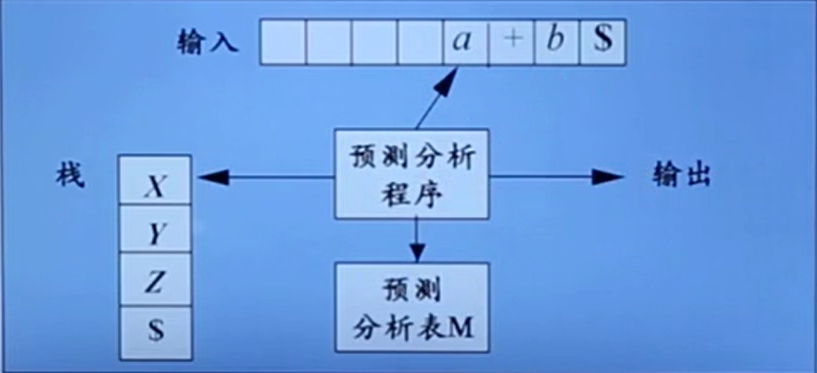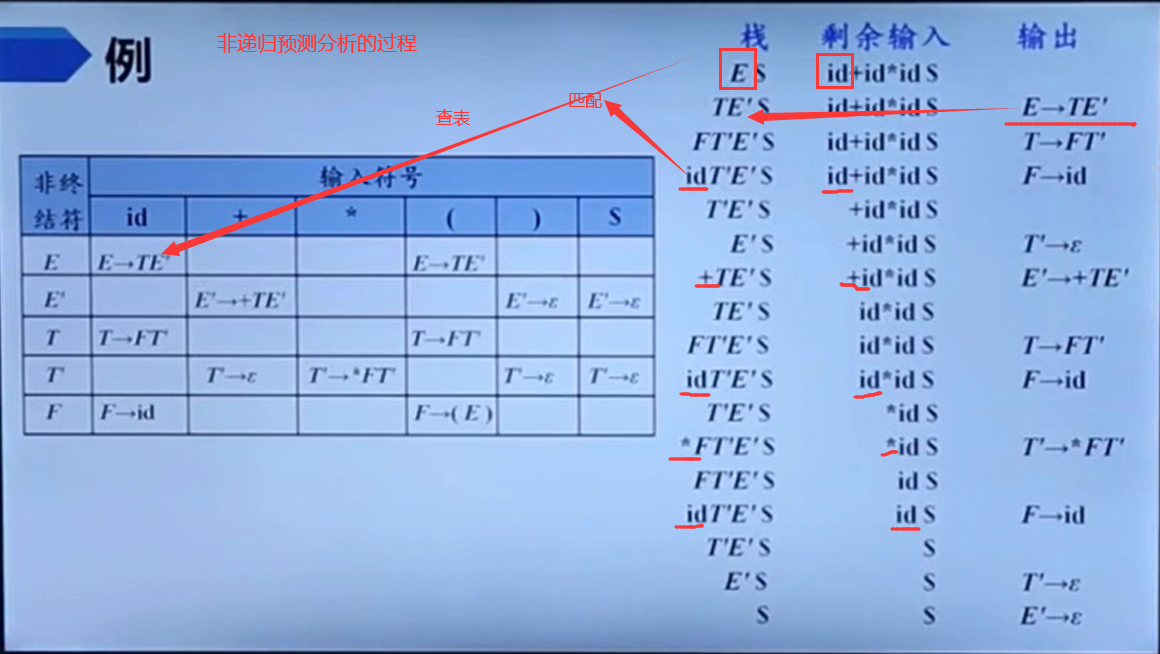预测分析中的错误检测
错误
栈顶的终结符和当前输入符号不匹配栈顶非终结符与当前输入符号在预测分析表对应项中的信息为空
处理
恐慌模式：
忽略输入中的一些符号，直到输入中出现由设计者选定的同步词法单元(synchromizing token)集合中的某个词法单元，同步集合的选取使得语法分析器能从实际遇到的错误中快速恢复，可以将FOLLOW(A)中的所有终结符放入非终结符A的同步记号集合  如果终结符在栈顶而不能匹配，一个简单的办法就是弹出此终结符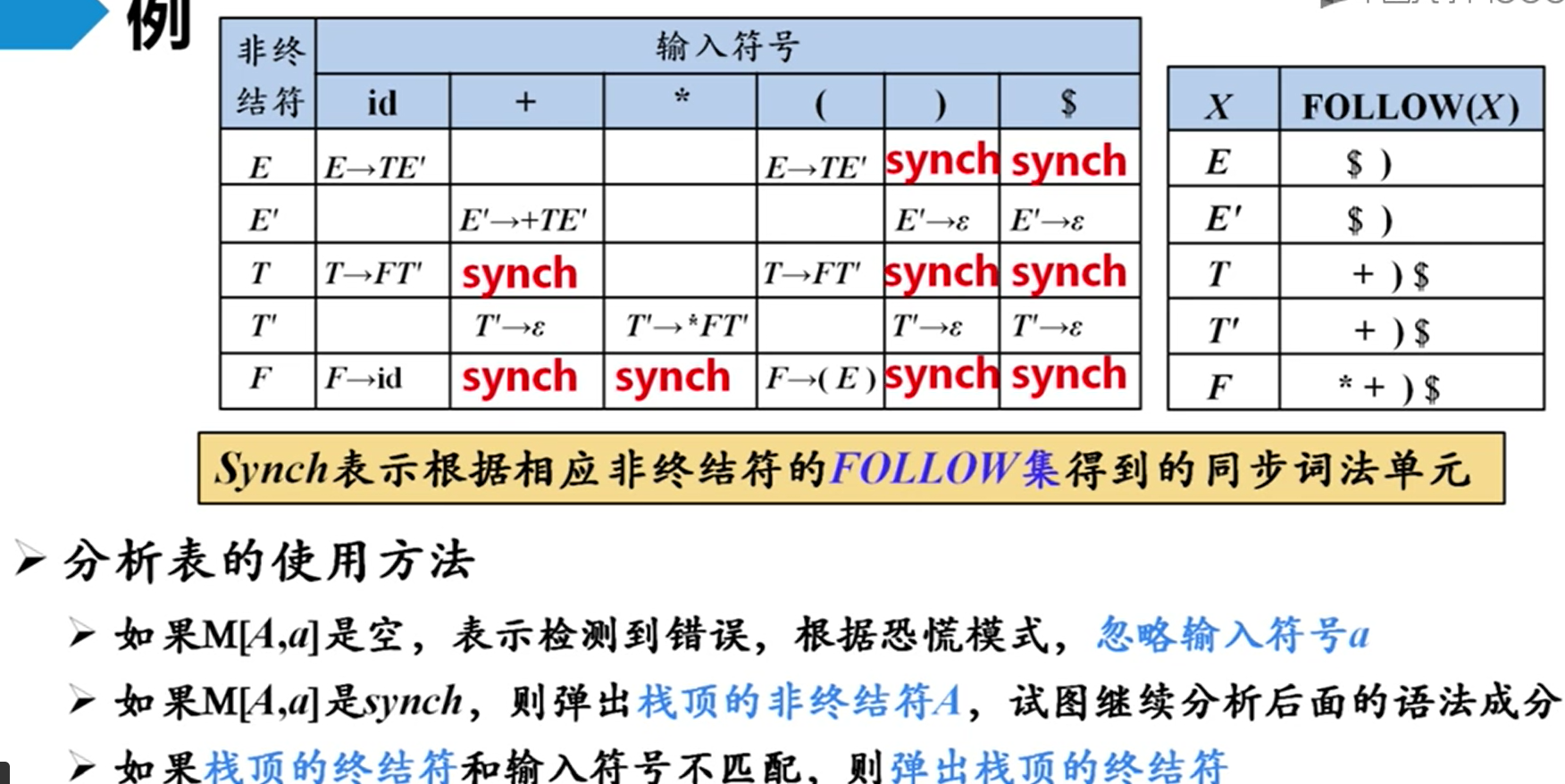自底向上的语法分析
分析树的底部(叶节点)向顶部(根节点)方向构造分析树将输入串w规约为文法开始符号S的过程自顶向下语法分析采用最左推导方式, 自底向上语法分析采用最左规约方式(反向构造最右推导)自底向上语法分析的通用框架是：移入-规约分析
移入-规约过程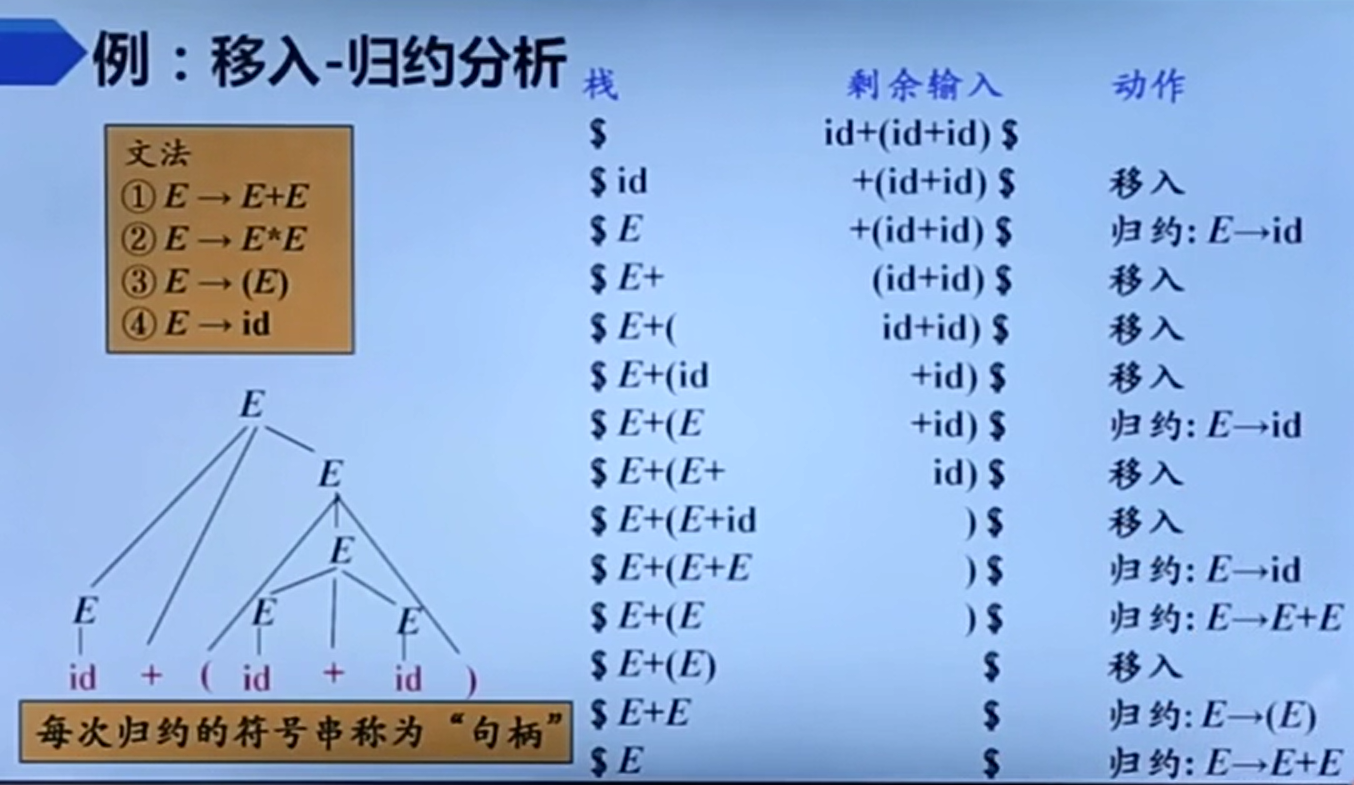堆栈内符号串 + 剩余输入 = 规范句型，规范句型相当于最右推导

移入-规约分析中存在的问题
每次规约的符号串是直接短语，造成错误的原因是错误的识别了句柄，也就是选错了产生式

直接短语：直接短语一定是产生式的右部，产生式的右部不一定是直接短语，并且是高度为二子树的边缘句柄：句型的最左直接短语

LR分析法概述
可以用LR文法分析的文法叫做LR文法，LR文法是最大的、可以构造出相应移入-规约语法分析器的文法类

L：对输入进行从左到右的扫描R：反向构造出一个最右推导序列LR(k) 需要向前查看k个输入符号的LR分析,一般k = 0 或者 k = 1
LR分析法的基本原理
自底向上分析的关键问题是什么？ 如何正确的识别句柄句柄是逐步形成的，用“状态” 表示句柄识别的进展程度 S—>bBB S—> •bBB (移进状态) S—>b•BB (待约状态) S—>bB•B (待约状态） S—>bBB• (规约状态)
LR分析器(自动机)的总体结构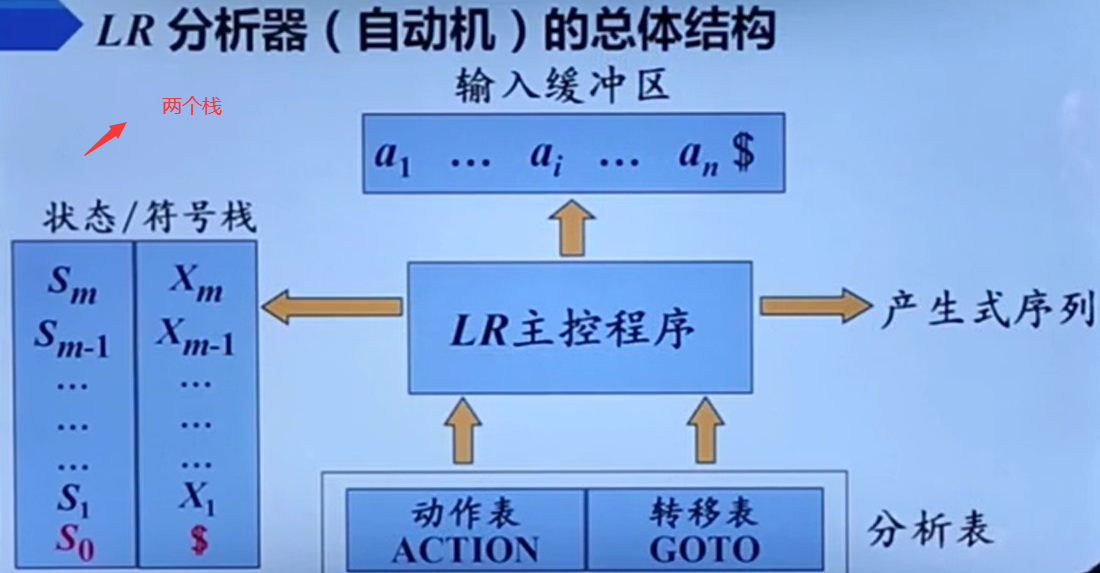LR分析表的结构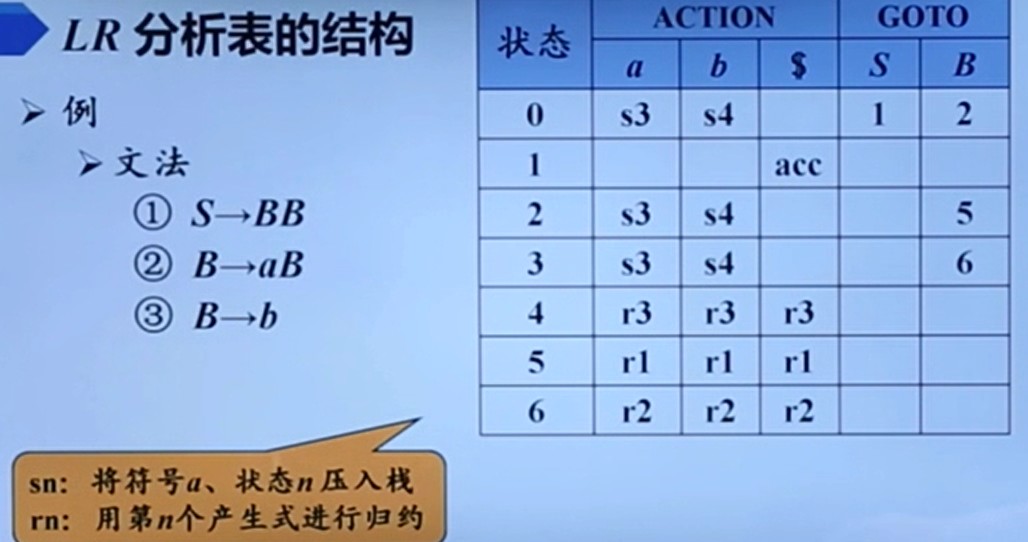LR(0)分析法
右部某位置标有圆点的产生式称为相应文法的一个LR(0)项目，一个产生式有k个符号，就会有k+1个项目

增广文法
就是引入一个符号，使文法开始符号只出现在一个产生式的左边，从而使得分析器只有一个接受状态

文法中的项目
初始项目：圆点在最左边规约项目：圆点在最右边后继项目：两个项目之间间隔一个圆点
等价项目
当圆点后面是一个非终结符的时候，就会存在等价项目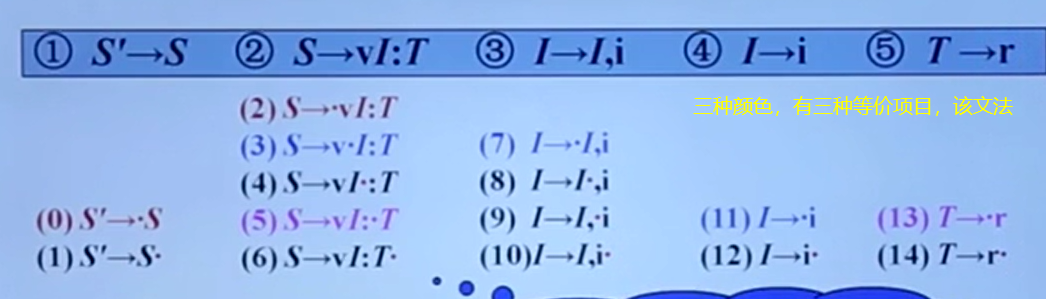项目集闭包
把所有的等价项目组成一个项目集(I),称为项目集闭包，每个项目集闭包对应着自动机的一个状态

LR(0)自动机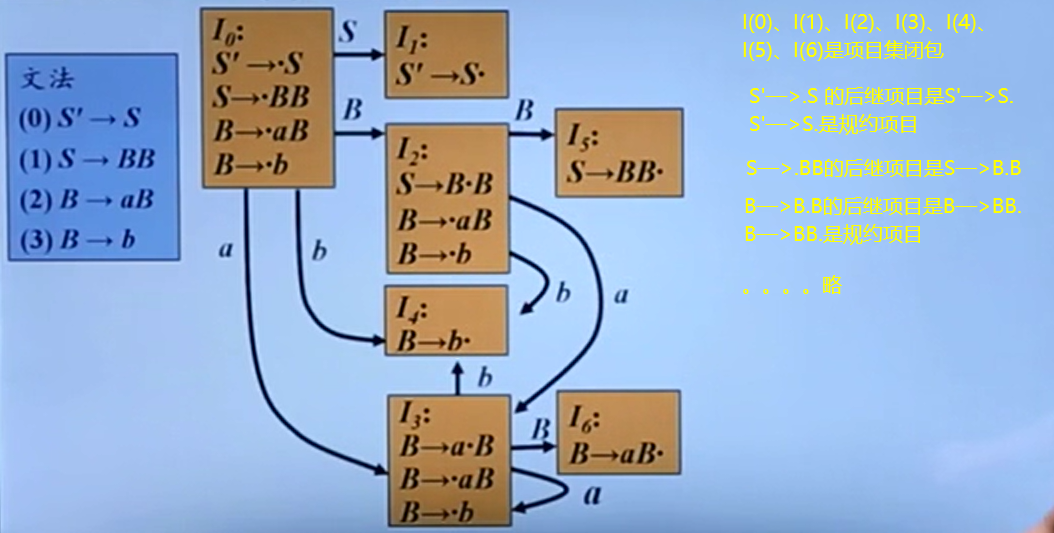LR(0)自动机构造LR(0)分析表
根据上图的自动机得来的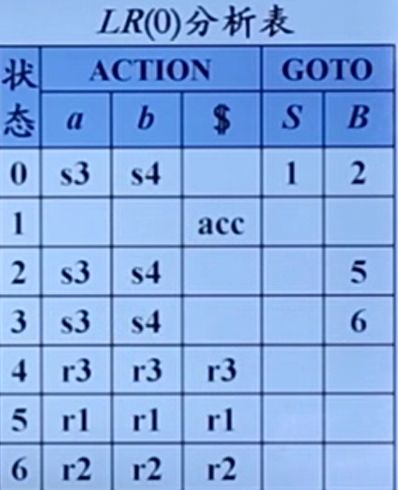LR(0)分析过程中的冲突
移进-规约冲突
无法确定是移进还是规约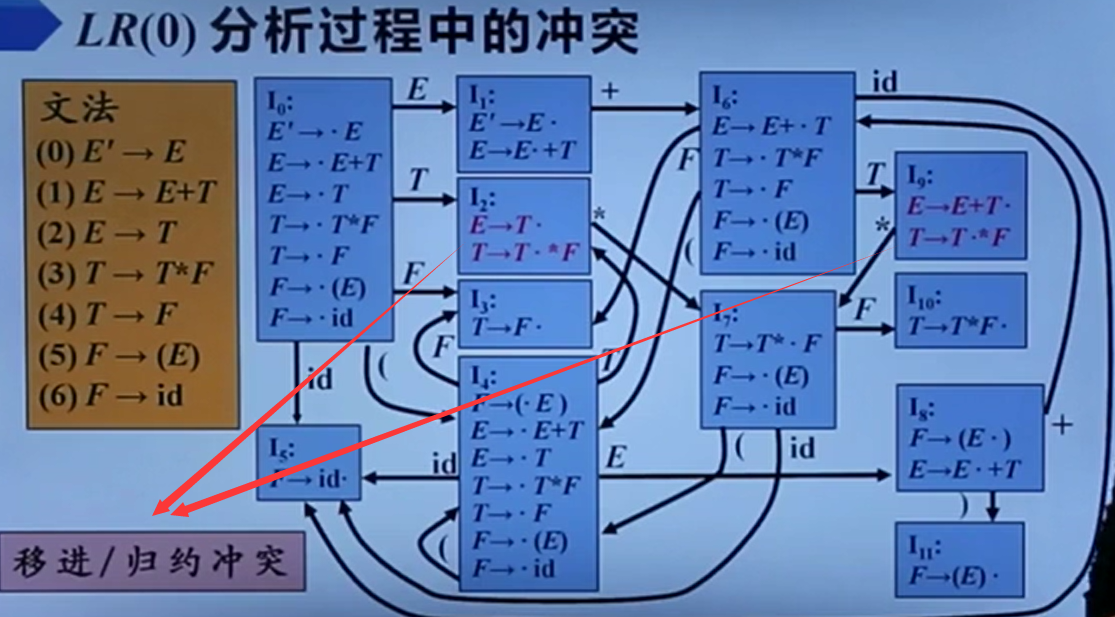规约-规约冲突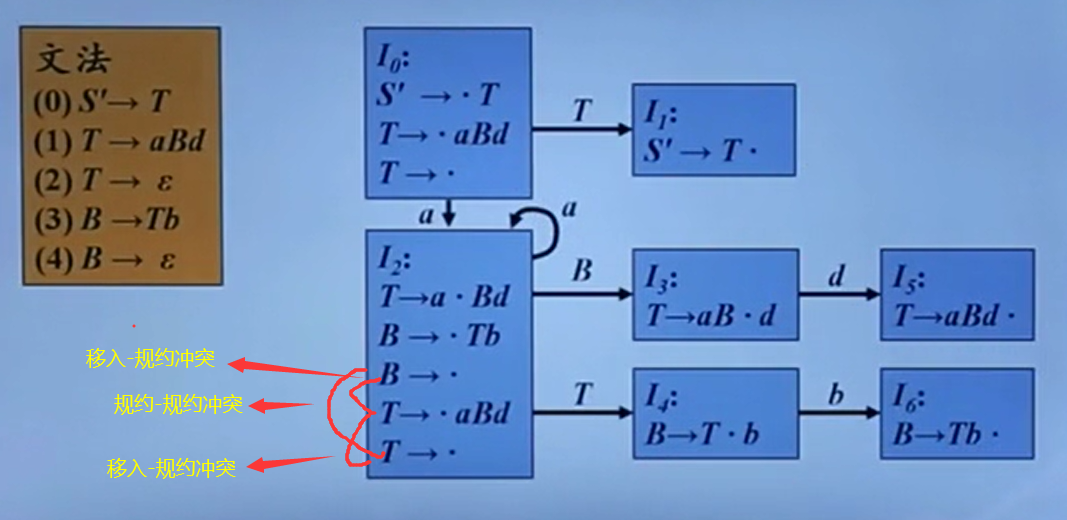没有这两种冲突的文法是LR(0)文法，不是所有的上下文无关文法都可以用LR(0)文法分析


SLR分析
为了解决LR(0)分析过程中的冲突的问题(也就是识别句柄的问题),出现了SLR分析，也叫做SLR(1)分析，S是Simple的意思，简单的LR(1)

SLR分析基本思想

需要使用到FOLLOW集，在不知道是否移进或者规约时,向后查看一个输入符号，根据FOLLOW集的定义是：该终结符后面可以的跟的终结符的集合。

看规约项目产生式左部的非终结符的FOLLOW集，如果下一个输入符号在FOLLOW集中就规约看移进项目的下一个终结符是和下一个输入符号一样，如果相同就移进详情看图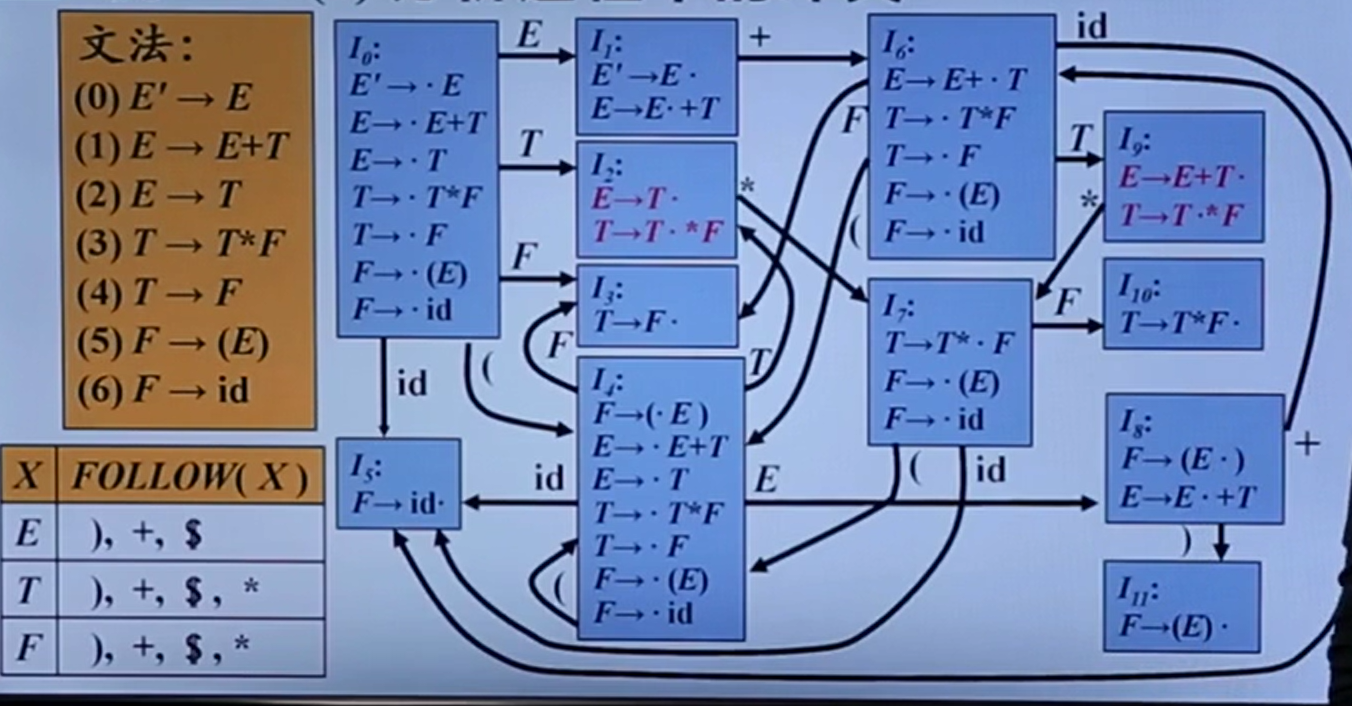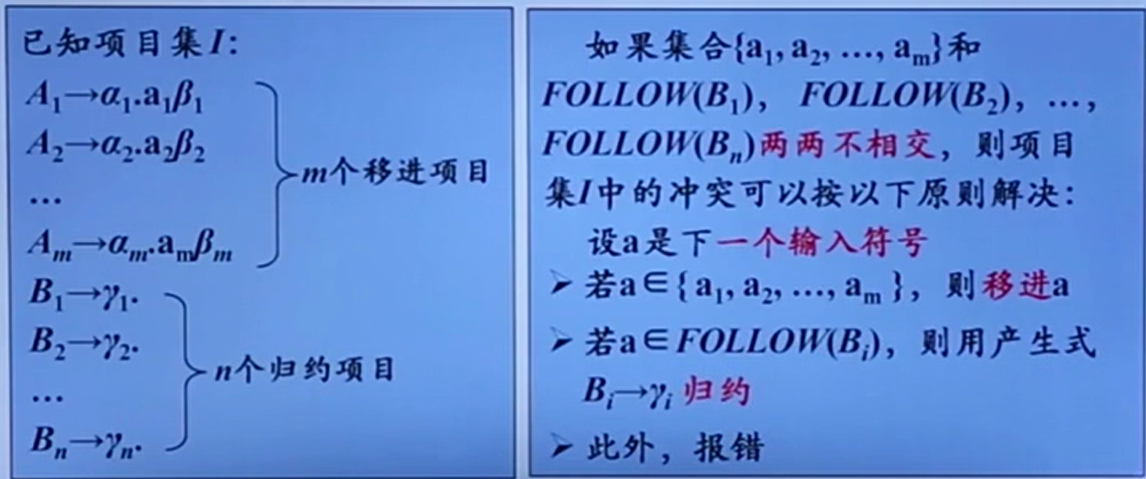例子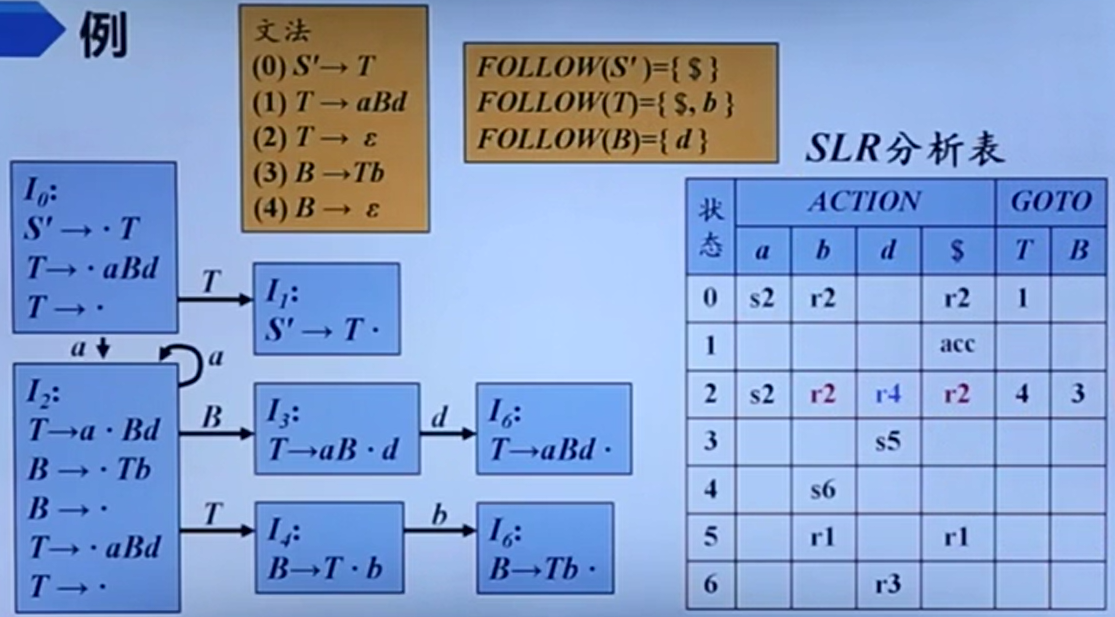SLR分析中的冲突
如果产生式符合SLR分析基本思想的两种规则，就无法判定到底是移进还是规约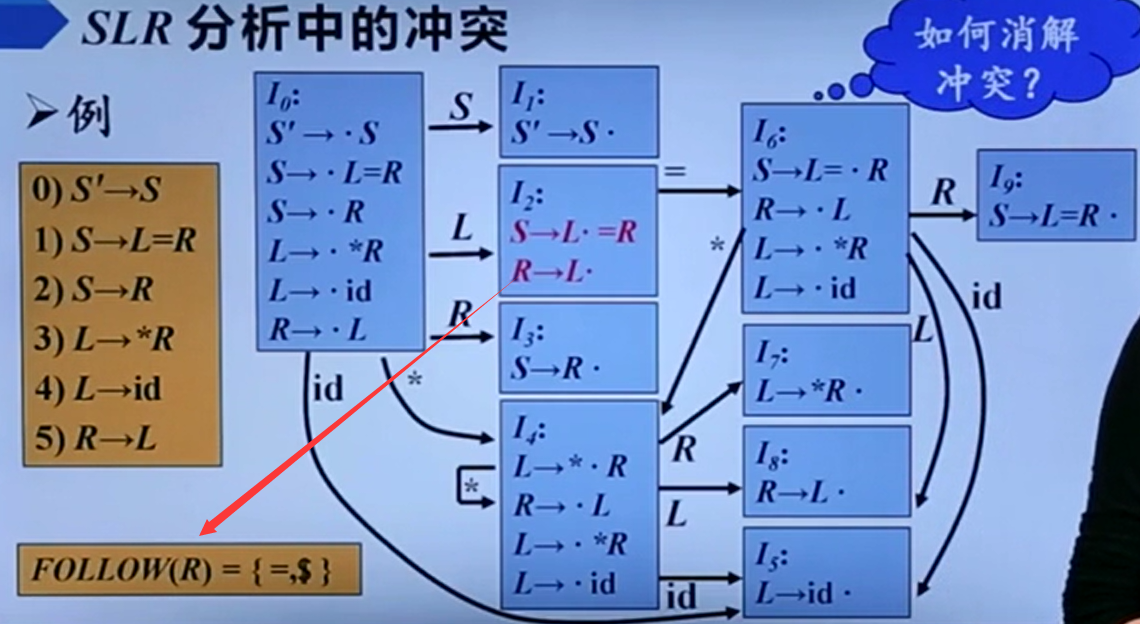LR(1)分析法
SLR使用FOLLOW集可以排除一些问题，但是不能解决所有问题

LR(1)分析法的提出
产生式A—>a规约时，在不同的位置，要求有不同的后继符号，在特定位置时，A的后继符号集合是FOLLOW(A)的子集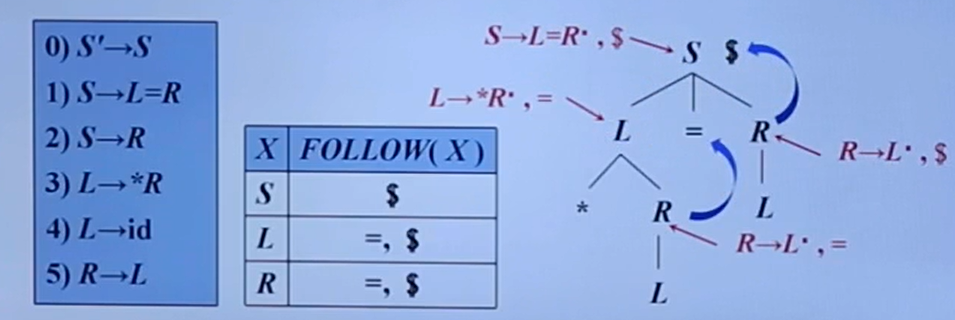LR(1)项目
将一个形式为[A—>α• β ,a]的项称为LR(1)项，其中A—>α β 是一个产生式，a是一个终结符(这里将$视为一个特殊的终结符)它表示在当前状态下，A后面必须紧跟终结符，称为该项的展望符LR(1)中的1指的是项的第二个分量的长度(就是向前查看一个符号)在形如[A—>α• β,a]且β≠ ε的项中，展望符a没有任何作用形如[A—>α•,a]的项在只有在下一个输入符号等于a时才可以安装A—>a进行规约，这样的a的集合总是FOLLOW(A)的子集，而且通常是一个真子集 LR(1)等价项目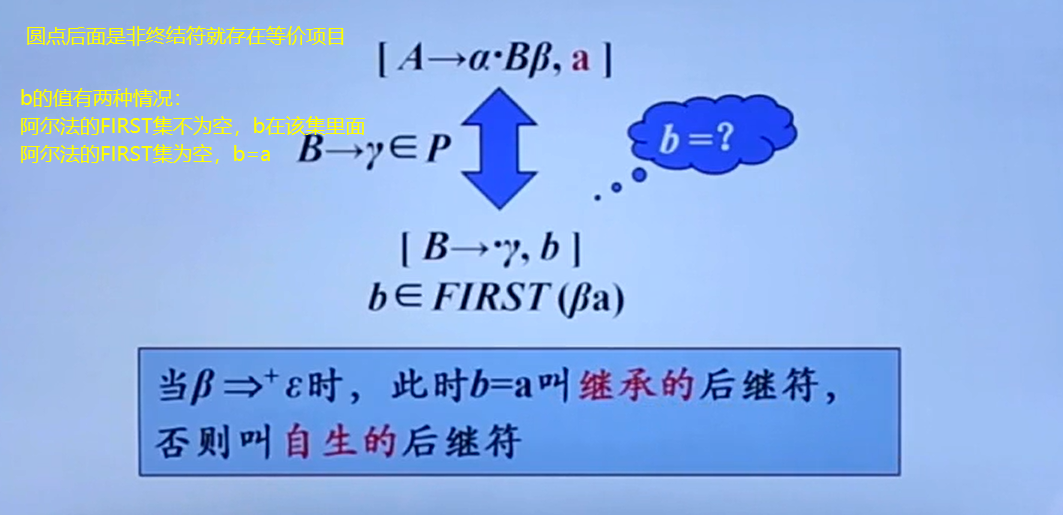LR(1)自动机 相比较LR(0)和SLR，多求了展望符，状态比较多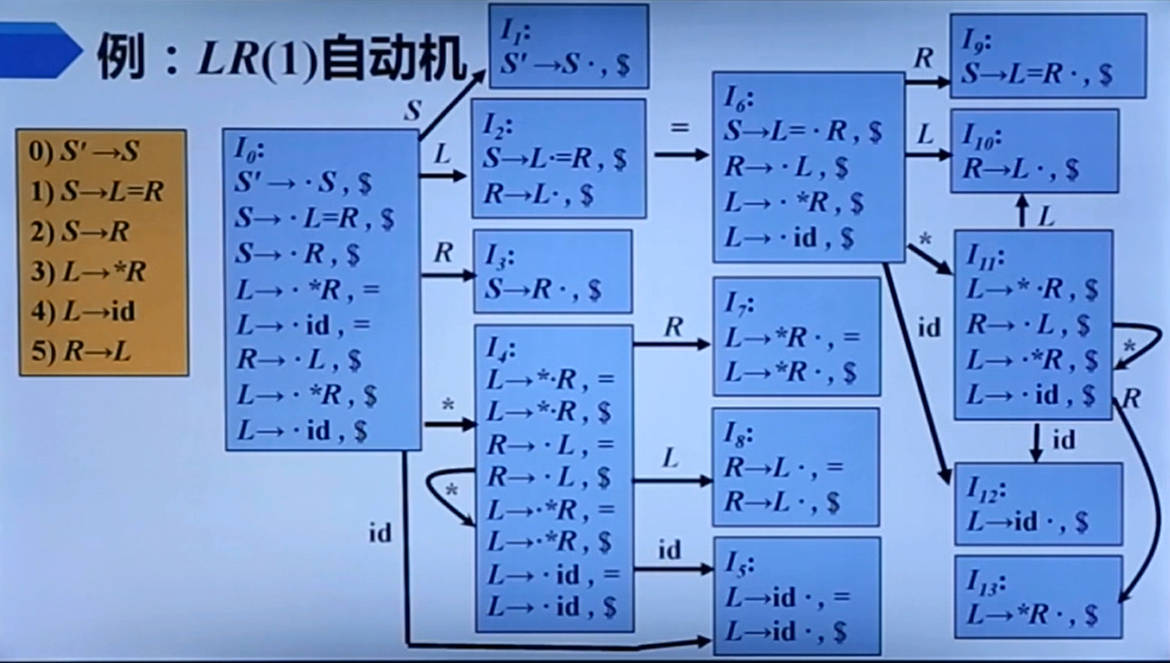LR(1)分析表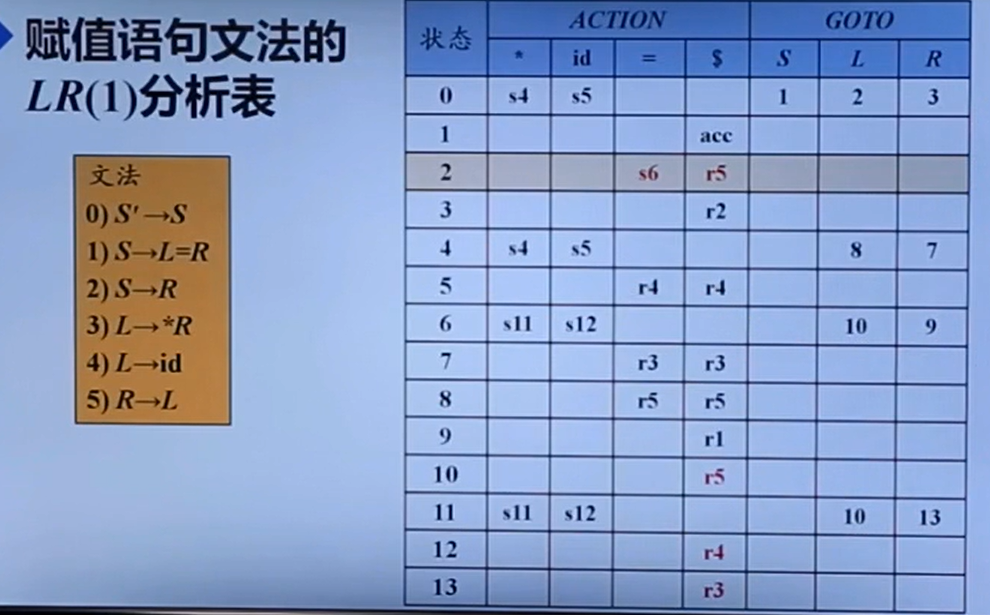展开全文• 四元组的形式展示出来： 文法G 定义为四元组G={Vn,Vt ,P,S} Vn ：非终结符集 Vt ：终结符集 P ：产生式集合（规则集合） S ：开始符号（识别符号) 文法类型： 文法类型分为四种：0型文法、1型文法、2型文法和3型... 根据文法规则，判断文法类型 1、实验要求 输入：文法规则 输出：文法类型 2、实验原理 文法规则： 以四元组的形式展示出来： 文法G 定义为四元组G={Vn,Vt ,P,S} Vn ：非终结符集 Vt ：终结符集 P ：产生式集合（规则集合） S ：开始符号（识别符号) 文法类型： 文法类型分为四种：0型文法、1型文法、2型文法和3型文法 0型文法：通俗的说，写出来的文法规则都输出0型文法1型文法：又称上下文有关文法。 满足条件：0型文法的基础上，右边字符个数不小于左边字符个数 例如：S->aBC a->Bb C->c2型文法：又称上下文无关文法。 满足条件：在1型文法的基础上，左部只有一个非终结符 例如：S->aBC B->b C->c3型文法：又称规范文法。 满足条件：在2型文法的基础上，产生式的右边字符数不超过2个且所有产生式要么都是左递归，要么都是右递归 例如：S->aB B->bC C->c 注： S->aB B->Cb C->c 不成立，因为字符串既有左递归，又有右递归 四种文法的关系可用如下图表示：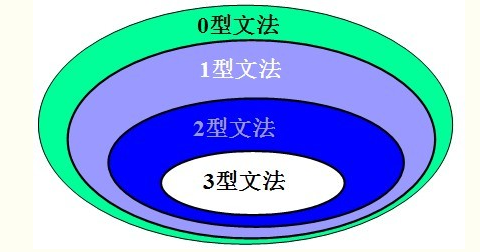3、实验步骤 从键盘上输入文法规则（字符串）对字符串进行按行比较，判断其所属的文法类型（先逐行判断文法类型1，然后再逐行判断文法类型2，最后在整个字符串判断文法类型3）显示出结果（文法类型） 4、实验过程 首先是进行结构体的构建，并且为了方便实用定义了全局变量 //结构体 struct Rule{ char str; int k;//记录局部文法类型 int left;//记录产生式左边长度 int right;//记录产生式右边长度 }r; int temp;//记录字符串行数 int level=0;//记录文法类型  主函数运到了5个调用函数，分别是输入板块、文法类型1判断板块、文法类型2判断板块、文法类型3判断板块和输出板块 //主函数 int main(){ Input();//输入文法规则 Transform1();//类型1判断 Transform2();//文法类型2判断 Transform3();//文法类型3判断 Output();//输出文法类型 return 0; }  在输入板块进行了文法规则的输入，一字符串的形式从键盘输入，存储到结构体中 //输入文法规则 void Input(){ int i,j; printf("输入文法规则：\n"); printf("大写字母为非终止符，小写字母为终止符，第一个产生式左边为开始符，结束用$表示\n");
for(i=0;i<10;i++){
scanf("%s",r[i].str);
j=0;
if(r[i].str[j]=='$') break; } temp=i; }  文法类型1板块时进行判断文法规则的类型是否符合类型1，符合之后才能进行类型2的判断 //判断文法类型1 void Transform1(){ int i,j; int length; for(i=0;i<temp;i++){ r[i].k=0; length=strlen(r[i].str);//字符串长度 r[i].left=0; for(j=0;r[i].str[j]!='-';j++)//左边长度 r[i].left++; r[i].right=length-r[i].left-2;//右边长度，减2表示减去字符'-'和'>' if(r[i].right>=r[i].left) r[i].k=1; } }  文法类型2板块时进行判断文法规则的类型是否符合类型2，符合之后才能进行类型3的判断 //判断文法类型2 void Transform2(){ int i,j,ss=0; for(i=0;i<temp;i++){//判断是否字符串是否都为类型1 if(r[i].k!=1) ss=1; } if(ss==0)//字符串都为类型1，进行类型2的判断 for(i=0;i<temp;i++){ j=0; if(r[i].left==1)//左边只有一个且是非终结符的类型1，则为类型2 if(r[i].str[j]>='A'&&r[i].str[j]<='Z') r[i].k=2; } level=1; }  文法类型3板块时进行判断文法规则的类型是否符合类型3 //判断文法类型3 void Transform3(){ int i,j,t,m,n=0,sum=0,ss=0; for(i=0;i<temp;i++){//判断是否字符串是否都为类型2 if(r[i].k!=2) ss=1; } if(ss==0){//字符串都为类型2，进行类型3的判断 level=2; for(i=0;i<temp;i++){ m=r[i].left+2;//产生式右边第一个字符 if(r[i].str[m]>='a'&&r[i].str[m]<='z') t=1; if(r[i].str[m]>='A'&&r[i].str[m]<='Z') t=0; sum+=t; if(r[i].right>2) n=1; } if(n==0)//保证产生式右边不超过2个字符 if(sum==temp|sum==0)//保证产生式右边是左递归或右递归 level=3; } }  7.在输出板块需要注意的是，不仅要将文法的类型输出，小编也将该四元组进行显示出来 //输出文法类型 void Output(){ int i,j,m=0,n=0; int t,p,ss; char S,V1,V2,Vn={},Vt={}; S=r.str;//开始符 for(i=0;i<temp;i++){ for(j=0;j<strlen(r[i].str);j++){ if(r[i].str[j]>='a'&&r[i].str[j]<='z') V1[m++]=r[i].str[j]; if(r[i].str[j]>='A'&&r[i].str[j]<='Z') V2[n++]=r[i].str[j]; } } t=0; for(i=0;i<m;i++){//终止符 ss=1; for(j=0;j<t;j++){ if(V1[i]==Vt[j]) ss=0; } if(ss==1) Vt[t++]=V1[i]; } p=0; for(i=0;i<n;i++){//非终止符 ss=1; for(j=0;j<p;j++){ if(V2[i]==Vn[j]) ss=0; } if(ss==1) Vn[p++]=V2[i]; } printf("该文法为第%d类型\n",level);//输出 printf("该文法的四元组为：\n"); printf("S：%c\n",S); printf("Vn="); for(i=0;i<p;i++) printf("%2c",Vn[i]); printf("\nVt="); for(i=0;i<t;i++) printf("%2c",Vt[i]); printf("\nP：\n"); for(i=0;i<temp;i++) printf("%s\n",r[i].str); }  5、源代码和截图 代码已经过调试，无编译错误 #include<stdio.h> #include<string.h> //结构体 struct Rule{ char str; int k;//记录局部文法类型 int left;//记录产生式左边长度 int right;//记录产生式右边长度 }r; int temp;//记录字符串行数 int level=0;//记录文法类型 //输入文法规则 void Input(){ int i,j; printf("输入文法规则：\n"); printf("大写字母为非终止符，小写字母为终止符，第一个产生式左边为开始符，结束用$表示\n");
for(i=0;i<10;i++){
scanf("%s",r[i].str);
j=0;
if(r[i].str[j]=='\$')
break;
}
temp=i;
}

//输出文法类型
void Output(){
int i,j,m=0,n=0;
int t,p,ss;
char S,V1,V2,Vn={},Vt={};
S=r.str;//开始符
for(i=0;i<temp;i++){
for(j=0;j<strlen(r[i].str);j++){
if(r[i].str[j]>='a'&&r[i].str[j]<='z')
V1[m++]=r[i].str[j];
if(r[i].str[j]>='A'&&r[i].str[j]<='Z')
V2[n++]=r[i].str[j];
}
}
t=0;
for(i=0;i<m;i++){//终止符
ss=1;
for(j=0;j<t;j++){
if(V1[i]==Vt[j])
ss=0;
}
if(ss==1)
Vt[t++]=V1[i];
}
p=0;
for(i=0;i<n;i++){//非终止符
ss=1;
for(j=0;j<p;j++){
if(V2[i]==Vn[j])
ss=0;
}
if(ss==1)
Vn[p++]=V2[i];
}
printf("该文法为第%d类型\n",level);//输出
printf("该文法的四元组为：\n");
printf("S：%c\n",S);
printf("Vn=");
for(i=0;i<p;i++)
printf("%2c",Vn[i]);
printf("\nVt=");
for(i=0;i<t;i++)
printf("%2c",Vt[i]);
printf("\nP：\n");
for(i=0;i<temp;i++)
printf("%s\n",r[i].str);
}

//判断文法类型3
void Transform3(){
int i,j,t,m,n=0,sum=0,ss=0;
for(i=0;i<temp;i++){//判断是否字符串是否都为类型2
if(r[i].k!=2)
ss=1;
}
if(ss==0){//字符串都为类型2，进行类型3的判断
level=2;
for(i=0;i<temp;i++){
m=r[i].left+2;//产生式右边第一个字符
if(r[i].str[m]>='a'&&r[i].str[m]<='z')
t=1;
if(r[i].str[m]>='A'&&r[i].str[m]<='Z')
t=0;
sum+=t;
if(r[i].right>2)
n=1;
}
if(n==0)//保证产生式右边不超过2个字符
if(sum==temp|sum==0)//保证产生式右边是左递归或右递归
level=3;
}
}

//判断文法类型2
void Transform2(){
int i,j,ss=0;
for(i=0;i<temp;i++){//判断是否字符串是否都为类型1
if(r[i].k!=1)
ss=1;
}
if(ss==0)//字符串都为类型1，进行类型2的判断
for(i=0;i<temp;i++){
j=0;
if(r[i].left==1)//左边只有一个且是非终结符的类型1，则为类型2
if(r[i].str[j]>='A'&&r[i].str[j]<='Z')
r[i].k=2;
}
level=1;
}

//判断文法类型1
void Transform1(){
int i,j;
int length;
for(i=0;i<temp;i++){
r[i].k=0;
length=strlen(r[i].str);//字符串长度
r[i].left=0;
for(j=0;r[i].str[j]!='-';j++)//左边长度
r[i].left++;
r[i].right=length-r[i].left-2;//右边长度，减2表示减去字符'-'和'>'
if(r[i].right>=r[i].left)
r[i].k=1;
}
}

//主函数
int main(){
Input();//输入文法规则
Transform1();//类型1判断
Transform2();//文法类型2判断
Transform3();//文法类型3判断
Output();//输出文法类型
return 0;
}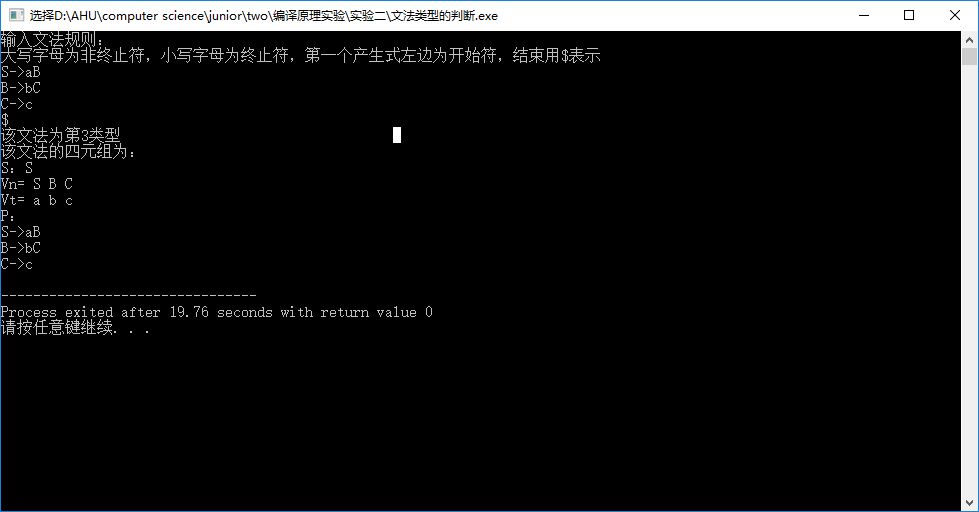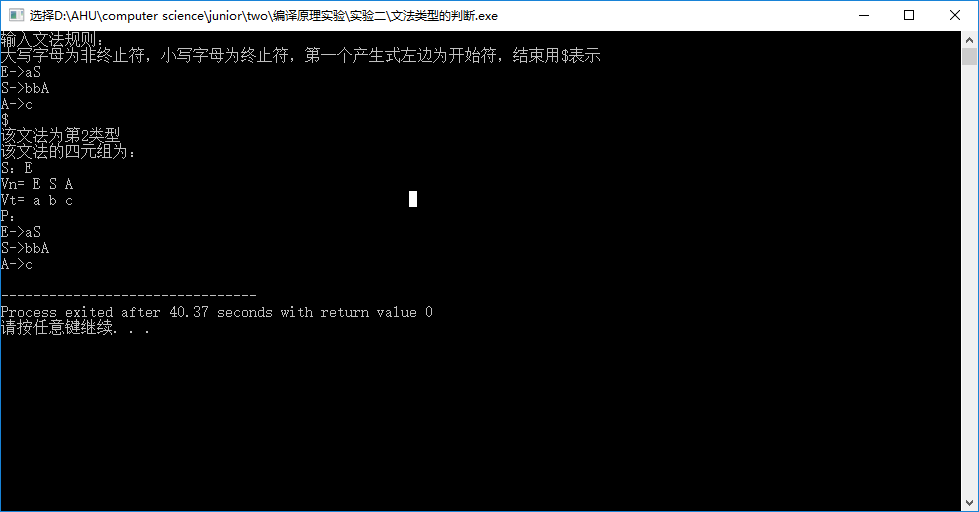展开全文编译原理
• 最近刚刚学编译原理，把对那几种文法罗列一下！   0型文法其中,至少含有一个非终结符，并且，表示终结符和非终结符的并集。 1型文法：又称为上下文有关文法， （1）：式子左边可以有多个字符，但必须有一个非...
• 乔姆斯基把方法分成四种类型，即0型、1型、2型和3型。 ... 0型文法 设G=（VN，VT，P，S），如果它的每个产生式α→β是...0型文法也称短语文法。一个非常重要的理论结果是：0型文法的能力相当于图灵机(Turing)。或...编译原理
• 文章目录自顶向下分析概述预测分析文法转换消除直接左递归消除间接左递归同一非终结符的多个候选式存在共同前缀：提取左公因子(Left Factoring )LL(1) 文法非终结符的后继符号集串首终结符集产生式A→α的可选集...
• Chomsky文法类型判断及文法化简 Chomsky文法类型判断及文法化简
• ## 文法分类

千次阅读 2018-05-30 19:27:24
首先先简单的介绍下两个概念 语言是由文法描述的,文法使用有限的规则... 文法分为0型文法、1型文法、2型文法、3型文法 0型文法只需要对α有要求 至少包含1个非终结符 1型文法在0型文法的基础上进一步的要...
• LR分析文法判断字符串应用语法是否正确，数据文法表示源程序，并且得到程序的执行结果。数据文法根据LR分析文法的句柄产生，是处理程序输入数据的产生式过程结构，所以称为数据文法。 数据文法的产生式称为数据产生...
• ## C语言文法

千次阅读 2015-01-18 01:24:31
• ## chomsky文法分类

千次阅读 2018-10-30 13:01:44
chomsky文法分类0123型 乔姆斯基把文法法分成四种类型，即0型、1型、2型和3型。这几种文法类型的概念一定要掌握，是一个非常重要的考点 0型文法 设G=(VN,VT,P,S)G=(V_N,V_T,P,S)G=(VN​,VT​,P,S)，如果它的每个产生...编译原理
• 今天这一份是英文文法语法类的题库，它包含3个表，表与表之间可以通过ID进行关联
• 首先定义一个产生式  α→β ... 0型文法，也称短语文法，α(​​​​​​​ ,)且至少含有一个非终结符，且β(​​​​​​​ ,)的闭包，一个非常重要的理论结果是，0型文法的能力相当于图灵...
• S_文法 条件： 每个产生式的右部都终结符开始 同一非终结符的各个候选式的首终结符都不同 局限性： 右部不能包含ε产生式 q_文法 引入SELECT集和FOLLOW集 q_文法中SELECT集的定义： 条件： 每个产生式的...编译原理
• 引用：＝＝＝＝＝＝＝＝＝＝＝＝＝＝＝＝＝＝＝＝S->...四种文法的判断非常简单，说到到，四种文法就是规定产生式的左和右边的字符的组成规则不同而已，其它的不能理解就不要去想了，你只要知道判断的时...
• 这本文法书是入门的文法书，读了这本书，再去读其它文法书，会比较好。英语要持之以恒，多背句子等。...